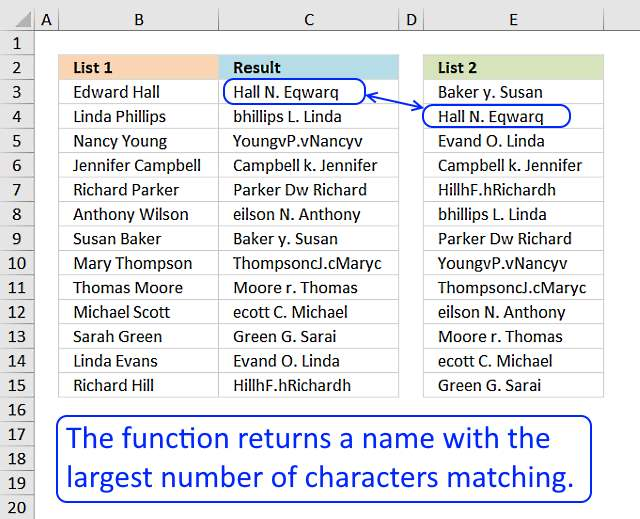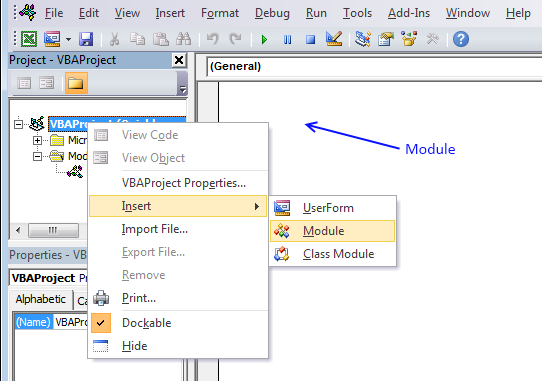Author: Oscar Cronquist Article last updated on January 15, 2019In this post I will describe a basic user defined function with better search functionality than the array formula in this post: Fuzzy vlookup.

The user defined function searches for a value with as many characters matching as possible. It is as simple as that.

I have added some serious misspellings randomly in List 2. 98 out of 100 names are found.

### User defined Function Syntax

SEARCHCHARS(lookup_value, tbl)

### Arguments

 lookup_value Required. The value you want to lookup. [tbl] Required. The range you want to search.

### Example

User defined function in cell C3:

=SEARCHCHARS(B3, \$E\$3:\$E\$101)

Copy cell C3 and paste it to the cells below, as far as needed.

### Excel vba

```'Name function and arguments
Function SearchChars(lookup_value As String, tbl_array As Range) As String
'Declare variables and types
Dim i As Integer, str As String, Value As String
Dim a As Integer, b As Integer, cell As Variant
'Iterste through each cell
For Each cell In tbl_array
'Save cell value to variable
str = cell
'Iterate through characters
For i = 1 To Len(lookup_value)
'Same character?
If InStr(cell, Mid(lookup_value, i, 1)) > 0 Then
'Add 1 to number in array
a = a + 1
'Remove evaluated character from cell and contine with remaning characters
cell = Mid(cell, 1, InStr(cell, Mid(lookup_value, i, 1)) - 1) & Mid(cell, InStr(cell, Mid(lookup_value, i, 1)) + 1, 9999)
End If
'Next character
Next i

a = a - Len(cell)
'Save value if there are more matching characters than before
If a > b Then
b = a
Value = str
End If

a = 0
Next cell
'Return value with the most matching characters
SearchChars = Value
End Function
```

### How to add the user defined function to your workbook1. Press Alt-F11 to open visual basic editor
2. Click Module on the Insert menu
3. Copy and paste the above user defined function
4. Exit visual basic editor

### Download Excel file through email

Enter your email address to receive the workbook. Note, an email will actually be sent to you.

* You will also get a weekly newsletter, unsubscribe anytime!